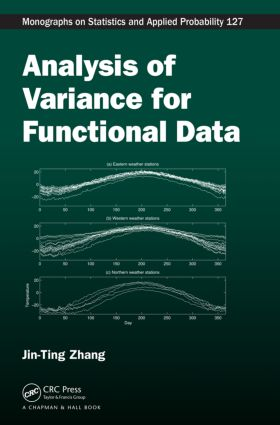Analysis of Variance for Functional Data

1st Edition

Chapman and Hall/CRC

412 pages | 80 B/W Illus.

Hardback: 9781439862735
pub: 2013-06-18
Currently out of stock
\$120.00
x
eBook (VitalSource) : 9780429070082
pub: 2013-06-18
from \$28.98

FREE Standard Shipping!

Description

Despite research interest in functional data analysis in the last three decades, few books are available on the subject. Filling this gap, Analysis of Variance for Functional Data presents up-to-date hypothesis testing methods for functional data analysis. The book covers the reconstruction of functional observations, functional ANOVA, functional linear models with functional responses, ill-conditioned functional linear models, diagnostics of functional observations, heteroscedastic ANOVA for functional data, and testing equality of covariance functions. Although the methodologies presented are designed for curve data, they can be extended to surface data.

Useful for statistical researchers and practitioners analyzing functional data, this self-contained book gives both a theoretical and applied treatment of functional data analysis supported by easy-to-use MATLAB® code. The author provides a number of simple methods for functional hypothesis testing. He discusses pointwise, L2-norm-based, F-type, and bootstrap tests.

Assuming only basic knowledge of statistics, calculus, and matrix algebra, the book explains the key ideas at a relatively low technical level using real data examples. Each chapter also includes bibliographical notes and exercises. Real functional data sets from the text and MATLAB codes for analyzing the data examples are available for download from the author’s website.

Reviews

"… a focused presentation of functional ANOVA and linear function-on-scalar regression problems using the ‘smooth first’ approach to estimation and inference. I would recommend this book to anyone interested in theoretical developments and hypothesis testing in this commonly encountered class of problems."

—Jeff Goldsmith, Journal of the American Statistical Association, March 2014

Introduction

Functional Data

Motivating Functional Data

Why Is Functional Data Analysis Needed?

Overview of the Book

Implementation of Methodologies

Nonparametric Smoothers for a Single Curve

Introduction

Local Polynomial Kernel Smoothing

Regression Splines

Smoothing Splines

P-Splines

Reconstruction of Functional Data

Introduction

Reconstruction Methods

Accuracy of LPK Reconstructions

Accuracy of LPK Reconstruction in FLMs

Stochastic Processes

Introduction

Stochastic Processes

x2-Type Mixtures

F-Type Mixtures

One-Sample Problem for Functional Data

ANOVA for Functional Data

Introduction

Two-Sample Problem

One-Way ANOVA

Two-Way ANOVA

Linear Models with Functional Responses

Introduction

Linear Models with Time-Independent Covariates

Linear Models with Time-Dependent Covariates

Ill-Conditioned Functional Linear Models

Introduction

Generalized Inverse Method

Reparameterization Method

Side-Condition Method

Diagnostics of Functional Observations

Introduction

Residual Functions

Functional Outlier Detection

Influential Case Detection

Robust Estimation of Coefficient Functions

Outlier Detection for a Sample of Functions

Heteroscedastic ANOVA for Functional Data

Introduction

Two-Sample Behrens-Fisher Problems

Heteroscedastic One-Way ANOVA

Heteroscedastic Two-Way ANOVA

Test of Equality of Covariance Functions

Introduction

Two-Sample Case

Multi-Sample Case

Bibliography

Index

Technical Proofs, Concluding Remarks, Bibliographical Notes, and Exercises appear at the end of most chapters.

Jin-Ting Zhang

Singapore, , Singapore